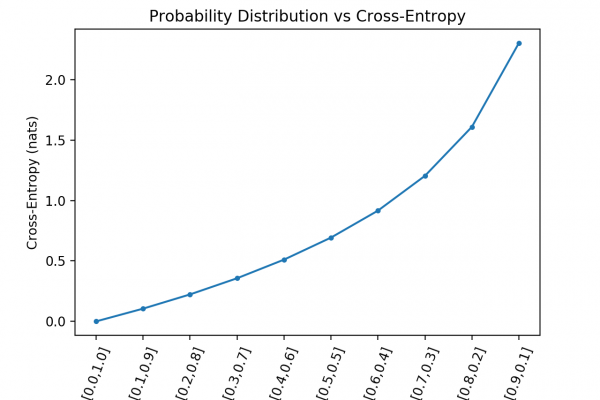# A Gentle Introduction to Cross-Entropy for Machine LearningLast Updated on December 20, 2019

Cross-entropy is commonly used in machine learning as a loss function.

Cross-entropy is a measure from the field of information theory, building upon entropy and generally calculating the difference between two probability distributions. It is closely related to but is different from KL divergence that calculates the relative entropy between two probability distributions, whereas cross-entropy can be thought to calculate the total entropy between the distributions.

Cross-entropy is also related to and often confused with logistic loss, called log loss. Although the two measures are derived from a different source, when used as loss functions for classification models, both measures calculate the same quantity and can be used interchangeably.

In this tutorial, you will discover cross-entropy for machine learning.

After completing this tutorial, you will know:

• How to calculate cross-entropy from scratch and using standard machine learning libraries.
• Cross-entropy can be used as a loss function when optimizing classification models like logistic regression and artificial neural networks.
• Cross-entropy is different from KL divergence but can be calculated using KL divergence, and is different from log loss but calculates the same quantity when used as a loss function.

Kick-start your